# WEEKNUM Function

Returns the week number of a specific date

## What is the WEEKNUM Function?

The WEEKNUM Function is an Excel DATE and TIME Function. It will return the week number of a specific date. The function will return an integer that represents a week number from 1 to 52 weeks of the year.

WEEKNUM is quite useful in financial analysis. Suppose we wish to determine the time required to complete a certain project, it can be used to remove weekends from the given timeframe. Thus, the function is particularly useful when planning and scheduling work for business projects.

### Formula

=WEEKNUM(serial_number,[return_type])

The WEEKNUM uses the following arguments:

1. Serial_number (required argument) – This is an Excel date for which we want to return the week number. When entering the argument, we should enter the date using the DATE function or as a result of other formulas or functions.
2. Return_type (optional argument) – This specifies which numbering system should be used and which day of the week should be treated as the start of the week.
• System 1 – The week containing January 1st is numbered week 1
• System 2 – The week containing the first Thursday of the year is numbered week 1

Possible values are:

Return_typeNumber returned
1 or omittedIt will take Sunday as the first day of week, so Sunday = 1, Monday = 2, ..., Saturday = 7. Uses numbering system 1
2It will take Monday as the first day of week, so Monday = 1, Tuesday = 2, ..., Sunday = 7. Uses numbering system 1
11Monday = 1, Tuesday = 2, ..., Sunday = 7. Uses numbering system 1
12Tuesday = 1, Wednesday = 2, ..., Monday = 7. Uses numbering system 1
13It will take Wednesday as the first day of week, so Wednesday = 1, Thursday = 2, ..., Tuesday = 7. Uses numbering system 1
14It will take Thursday as first day of week, so Thursday = 1, Friday = 2, ..., Wednesday = 7. system 1
15It shall take Friday as first day of week so, Friday = 1, Saturday = 2, ..., Thursday = 7. Uses numbering system 1
16It will take Saturday as the first day of week, Saturday = 1, Sunday = 2, ..., Friday = 7. Uses numbering system 1
17Sunday = 1, Monday = 2, ..., Saturday = 7. Uses numbering system 1
21Week runs from Monday to Sunday. Uses numbering system 2

### A few notes about the WEEKNUM Function

1. If we omit the return_type argument, it will take the default value of 1.
2. MS Excel stores dates as sequential serial numbers so they can be used in calculations. By default, January 1, 1900 is serial number 1, and January 1, 2009 is serial number 39448 because it is 39,448 days after January 1, 1900. Excel interprets text representations of dates differently, depending on the date settings on your computer. Therefore, the serial_number argument for the WEEKDAY function should be entered as either:
1. A reference to a cell containing a date
2. A date returned from another function or formula

### How to use the WEEKNUM Function in Excel?

WEEKNUM is a built-in function that can be used as a worksheet function in Excel. To understand the uses of the function, let’s consider a few examples:

#### Example 1

Suppose we are given the following dates. Let’s see how we can get the week number from the dataset.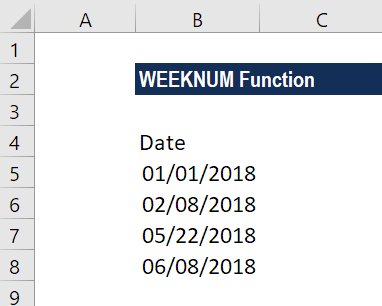The formula to use will be: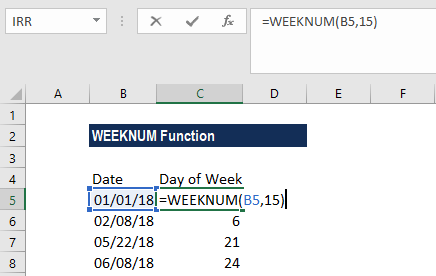Here, we took the return_type argument as 15, which means it will take Friday as day 1 of the week.

We get the results below: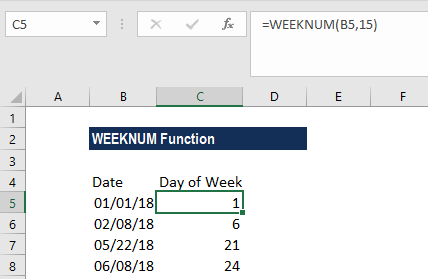#### Example 2

We can turn a week number into a date using the WEEKNUM function. Suppose we are given the data below: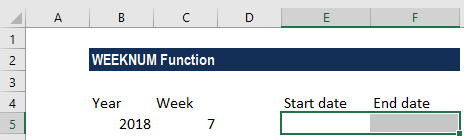For start_date, the formula to use will be: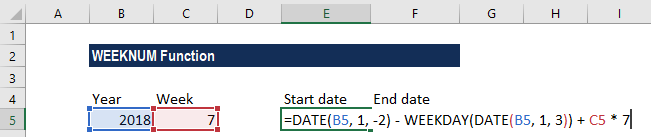It calculates the date of the last Monday in the previous year. C5*7 adds the number of weeks multiplied by 7 (the number of days in a week) to get the Monday (start date) of the week in question.

We get the result below: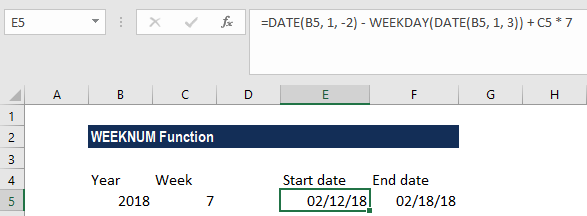For the end date, we will use the following formula: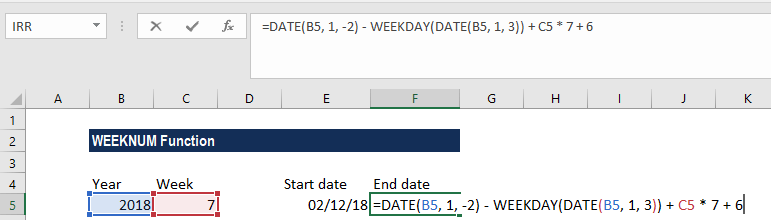We get the result below: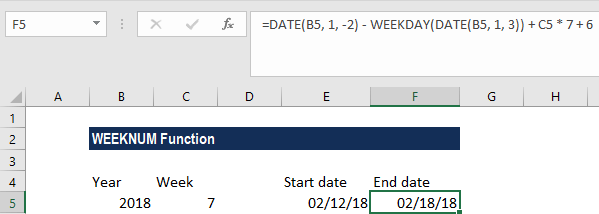The formula is based on the ISO week date system, where the week starts on Monday and the week containing the 1st Thursday of the year is considered week 1.

### Common errors in WEEKNUM Function

1. #VALUE! error – Occurs when either the given serial_number is non-numeric or cannot be recognized as a valid date.
2. #NUM! error – Occurs when:
1. The given return_type argument is not the value permitted by the function.
2. The given serial_number argument is numeric but is out of the range for the current date base.

Thanks for reading CFI’s guide to the Excel WEEKNUM function. By taking the time to learn and master these functions, you’ll significantly speed up your financial modeling. To learn more, check out these additional CFI resources:

• Excel Functions for Finance